﻿ 船用冷凝器真空专家控制系统研究
 舰船科学技术2016, Vol. 38Issue (5): 101-104PDF

Research on the expert control system of vacuum for the marine condenser
GONG Xian, SUN Jian-hua, ZOU Hai
Wuhan Second Ship Design and Research Institute, Wuhan 430205, China
Abstract: In order to relax the unfortunate effect which the variation of cooling water temperature plays on the system of condenser vacuum controla mathematic model of a marine condenser is presented according to its working principle and its structure features. An expert control system of condenser vacuum is designed. Simulink software is used for the simulation of the control system. In the simulation, the load of condenser and the temperature of cooling water changes separately. Result shows that the condenser vacuum is kept invariant by the expert control systemIt has referring significance for control system design of condenser vacuum.
Key words: condenser     vacuum     expert Control
0 引 言

1 船用冷凝器数学模型 1.1 假设条件

1）在壳侧的蒸汽区，认为是定压凝结，在定压的条件下凝结蒸汽温度不变；

2）忽略管子蓄热，视管壁温度与蒸汽温度相等；

3）由于蒸汽体积远大于热井冷凝水体积，可视为蒸汽体积等于冷凝器体积且为定值；

4）不考虑冷凝器向环境散热。

1.2 冷凝器模型 1.2.1 冷凝器壳侧

1）蒸汽凝结过程

 $\frac{\text{d}}{\text{d}\tau }\left( {{m}_{s}}{{h}_{s}} \right)=\sum\limits_{i}{{{G}_{i}}{{h}_{i}}}-{{q}_{n}}{{h}_{w}}-{{q}_{c}}{{h}_{c}}-Q,$ (1)
 $V\frac{\text{d}{{\rho }_{s}}}{\text{d}\tau }=\sum\limits_{i}{{{G}_{i}}}-{{q}_{n}}-{{q}_{c}}.$ (2)

 $Q=A\alpha \frac{{{t}_{ou}}-{{t}_{in}}}{\ln \frac{{{t}_{s}}-{{t}_{in}}}{{{t}_{s}}-{{t}_{ou}}}}.$ (3)

2）蒸汽和空气分压过程

 ${{p}_{k}}={{p}_{s}}+{{p}_{a}},$ (4)
 $\frac{\text{d}{{p}_{s}}}{\text{d}t}=\frac{\text{d}{{M}_{s}}}{\text{d}t}\cdot \frac{{{R}_{s}}\left( {{t}_{s}}+273 \right)}{V},$ (5)
 $\frac{\text{d}{{p}_{a}}}{\text{d}t}=\frac{\text{d}{{M}_{a}}}{\text{d}t}\cdot \frac{{{R}_{a}}\left( {{t}_{a}}+273 \right)}{V},$ (6)

1.2.2 冷凝器管侧

 $Q-D{{C}_{p}}\left( {{t}_{ou}}-{{t}_{in}} \right)={{M}_{w}}{{C}_{p}}\frac{\text{d}{{t}_{ou}}}{\text{d}\tau }.$ (7)

2 专家控制系统设计与仿真验证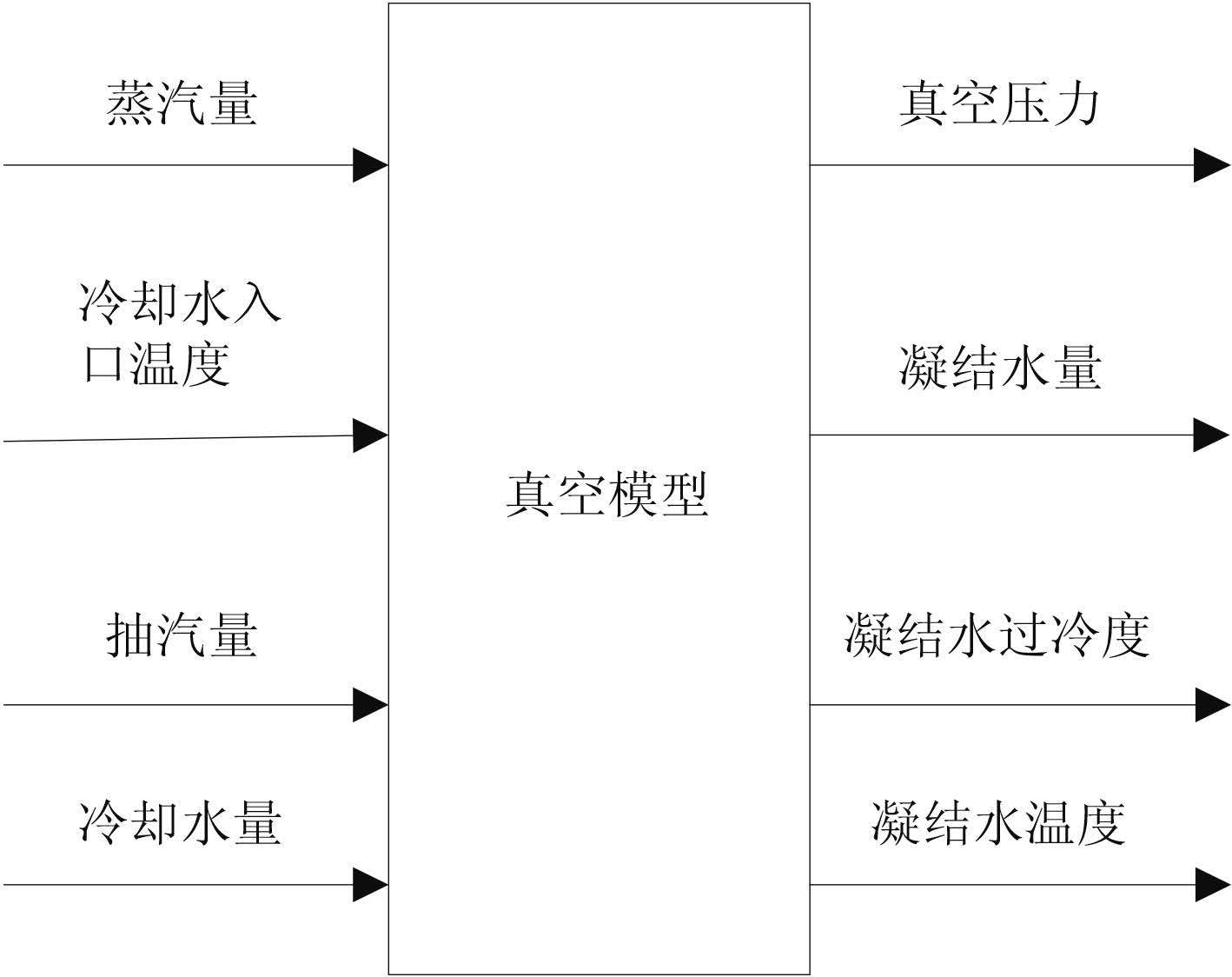图 1 凝器仿真模型结构图 Fig. 1 The simulation structure block of condenser model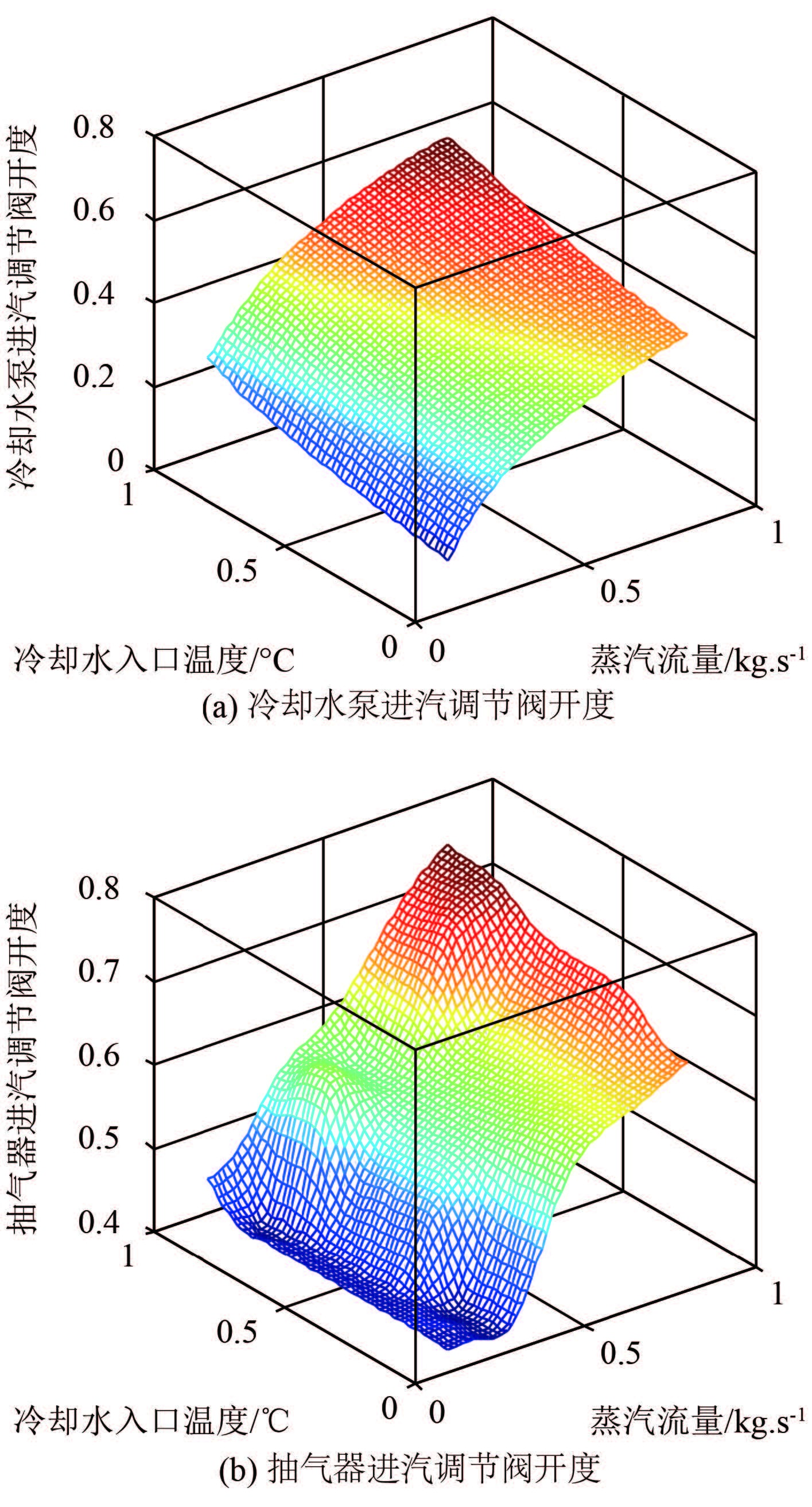图 2 真空控制专家知识图形化表示 Fig. 2 The graphical expert knowledge of vacuum control system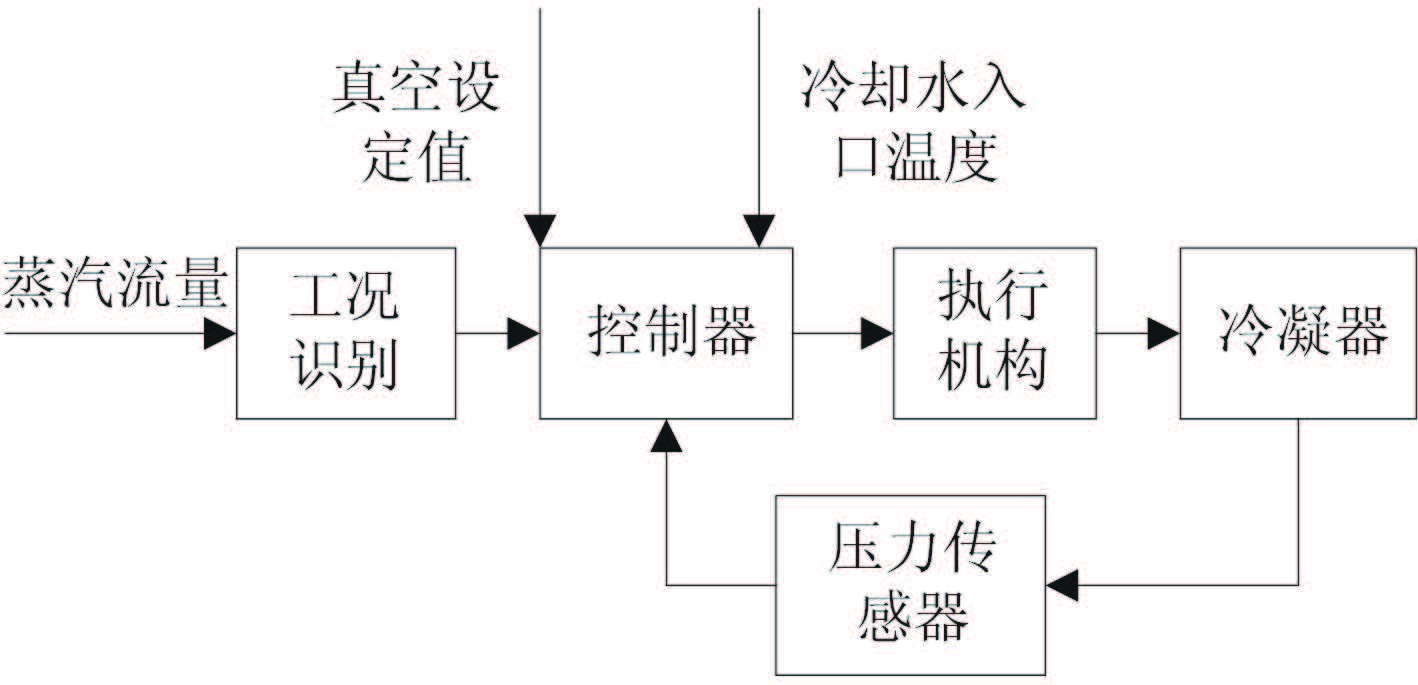图 3 冷凝器真空控制原理图 Fig. 3 Diagram of the condenser vacuum control principle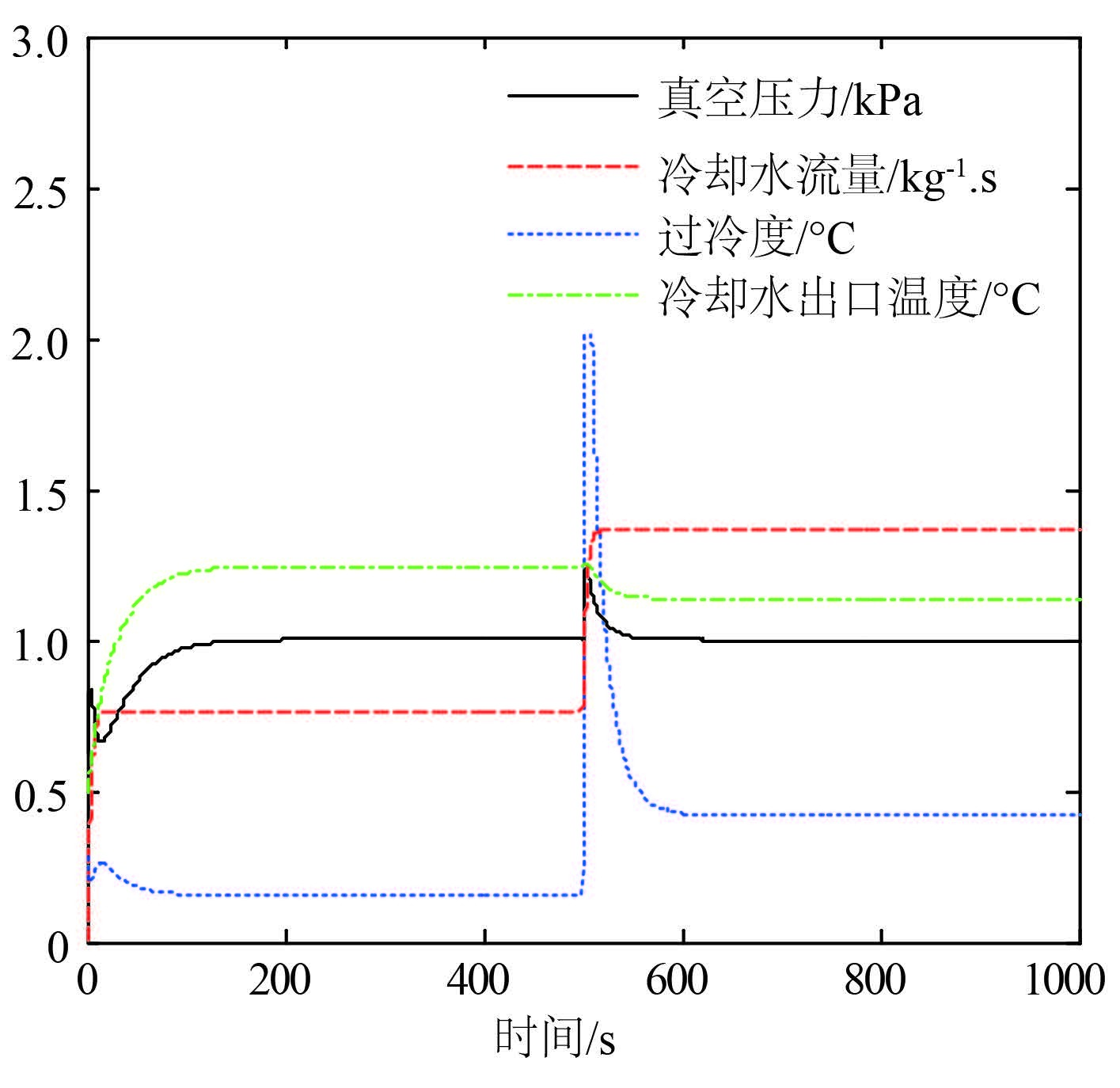图 4 变负荷仿真实验结果 Fig. 4 The simulation results of changing load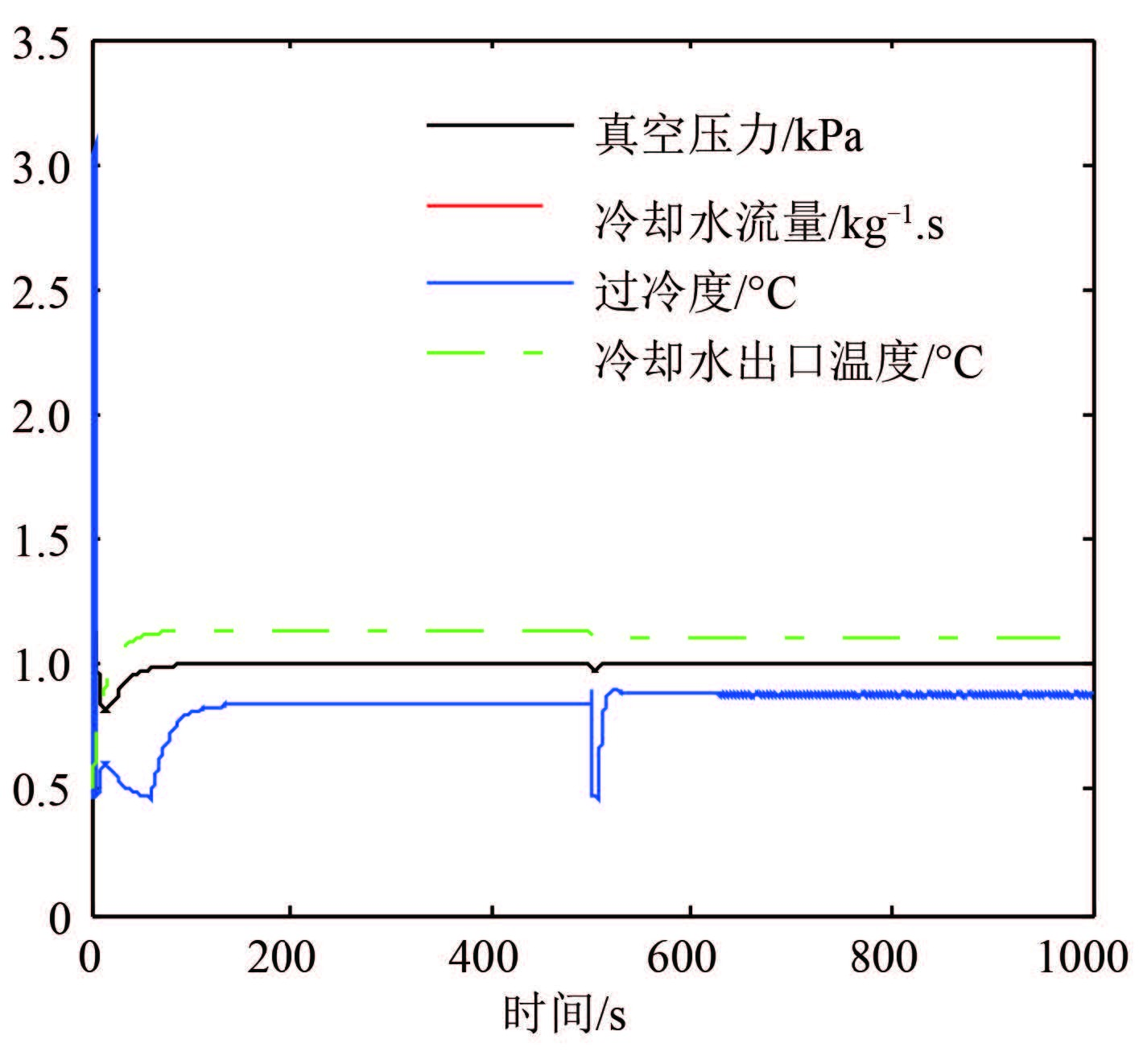图 5 变冷却水入口温度仿真实验结果 Fig. 5 The simulation results of changing cooling water temperature

3 结 语

  尹炜.船用凝汽系统动态建模及控制方法研究[D].哈尔滨:哈尔滨工程大学, 2008:12-22. YIN Wei. Research on dynamic modeling and control method of marine condensation-steam system[D]. Harbin:Harbin Engineering University, 2008:12-22. http://cdmd.cnki.com.cn/Article/CDMD-10217-2009059289.htm  朱东保, 马俊, 焦晓亮, 等. 船舶蒸汽动力装置冷凝器动态模型研究[J]. 节能技术 , 2010, 28 (6) :489–493. ZHU Dong-bao, MA Jun, JIAO Xiao-liang, et al. Condenser modeling research for ship steam power plants[J]. Energy Conservation Technology , 2010, 28 (6) :489–493.  张永生, 马运义, 唐滢, 等. 船用凝汽器的数学模型与动态仿真[J]. 舰船科学技术 , 2010, 32 (10) :101–103. ZHANG Yong-sheng, MA Yun-yi, TANG Ying, et al. Mathematic model of a marine condenser and the dynamic simulation[J]. Ship Science and Technology , 2010, 32 (10) :101–103.  倪何, 程刚, 孙丰瑞. 某型舰用冷凝器动态数学模型的建立和应用[J]. 计算机仿真 , 2007, 24 (7) :337–341. NI He, CHEN Gang, SUN Feng-rui. Application of a dynamic mathematic model of a naval condenser[J]. Computer Simulation , 2007, 24 (7) :337–341.  史觊, 孙建华, 付明玉, 等. 一种新型船舶核动力装置冷凝器模型与动态仿真方法[J]. 哈尔滨工程大学学报 , 2001, 22 (4) :8–11. SHI Ji, SUN Jian-hua, FU Ming-yu, et al. New model of con-denser for shipborne nuclear power unit and dynamic simulation[J]. Journal of Harbin Engineering University , 2001, 22 (4) :8–11.  张永生, 马运义. 船用冷凝器的真空与凝水过冷度控制系统研究[J]. 舰船科学技术 , 2011, 33 (3) :62–64. ZHANG Yong-sheng, MA Yun-yi. Research on the control system of vacuum and condensate subcooling degree for the marine condenser[J]. Ship Science and Technology , 2011, 33 (3) :62–64.  万华庆, 姚涌涛, 潘艳, 等. 凝汽器真空-凝水过冷度机理分析及其控制系统研究[J]. 舰船科学技术 , 2008, 30 (1) :80–83. WAN Hua-qing, YAO Yong-tao, PAN Yan, et al. Research of condensate depression and it's control system[J]. Ship Science and Technology , 2008, 30 (1) :80–83.  张伟, 边信黔, 夏国清, 等. 基于规则的冷凝器压力系统协调控制研究[J]. 热能动力工程 , 2009, 24 (2) :188–191. ZHANG Wei, BIAN Xin-qian, XIA Guo-qing, et al. Study of the rules-based coordinated control of a condenser pressure system[J]. Journal of Engineering for Thermal Energy and Power , 2009, 24 (2) :188–191.  余天星, 单军, 王鹏. 汽轮机凝汽器真空控制系统设计[J]. 控制工程 , 2006, 13 (S) :89–92. YU Tian-xing, SHAN Jun, WANG Peng. Design of the vacu-um control system of the steam turbine condenser[J]. Control Engineering of China , 2006, 13 (S) :89–92.  张琴舜, 邹文进, 沈秀中, 等. 冷凝器动态数学模型和仿真研究[J]. 计算机工程 , 2001, 27 (2) :186–187. ZHANG Qin-shun, ZOU Wen-jin, SHEN Xiu-zhong, et al. Modularization and simulation of condenser[J]. Computer Engineering , 2001, 27 (2) :186–187.